# Count Unique Values in a Range in Excel & Google Sheets

In this tutorial, we will demonstrate how to count unique values in a range in Excel and Google Sheets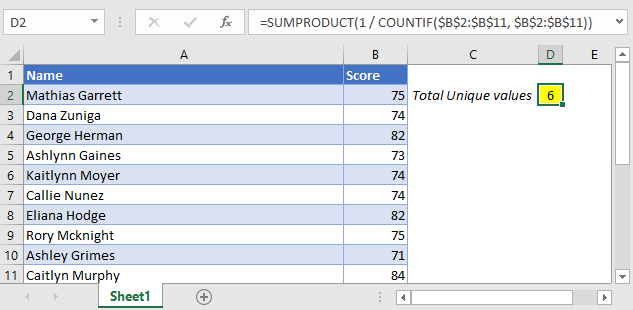## Count Unique Values in a Range with SUMPRODUCT and COUNTIF

The general formula we will use is this:

``````=SUMPRODUCT(1/COUNTIF(Range, Range))
``````

As you can see in the above formula, we are combining the  COUNTIF function with the SUMPRODUCT function.

``=SUMPRODUCT(1/COUNTIF(B2:B11, B2:B11))``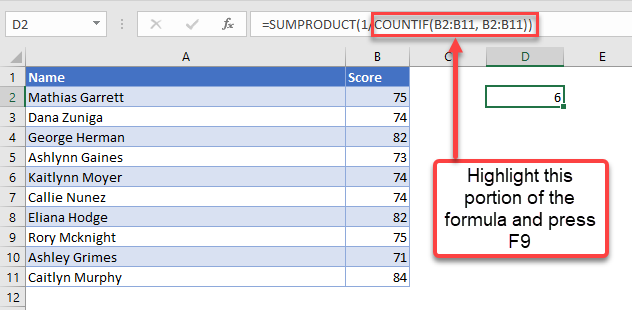## The COUNTIF Function

Let’s break down each section of the formula to understand it.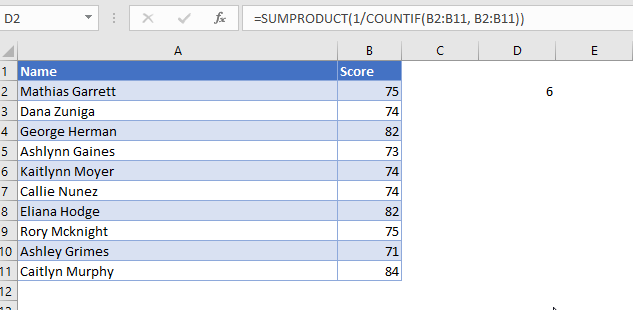As you can see above, you can do this by highlighting the COUNTIF portion of the formula and pressing F9.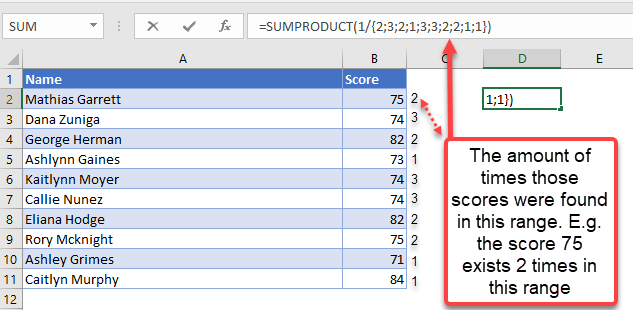The COUNTIF section of the formula calculates the amount of times that each value shows up in this range. In this case, the score of 75 exists in this range in two cells, the score of 74 exists in three cells, the score of 82 exists in two cells and so on.

Putting these values over a numerator of 1 converts these values into reciprocal functions. Let’s use the numbers 75 and 84 as examples. The number 75 came up twice which means that the reciprocal function of this is 1 / 2 or 0.5. The number 84 came up once which means the reciprocal function of this is 1 / 1 or just 1.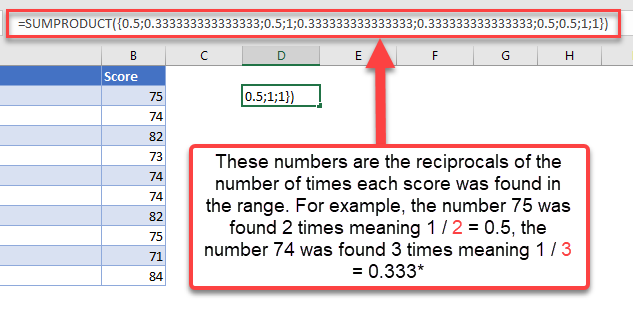The SUMPRODUCT portion of the formula adds all these reciprocal values together. Going back to the example of 75 which had a reciprocal of 1 / 2 (or 0.5), this multiplied by two (since it came up twice) means we end up with 1. The number 84 had a reciprocal of 1 / 1 (or 1) which multiplied by one (since it only came up once) means we end up with 1.

The combination of a reciprocal function and COUNTIF with the SUMPRODUCT function allows us to get a value of 1 for each unique value.

### Count unique values in a range with UNIQUE (Office 365)

If you have Office 365, you can use the following formula:

``=COUNT(UNIQUE(Range))``

## Count unique values in a range in Google Sheets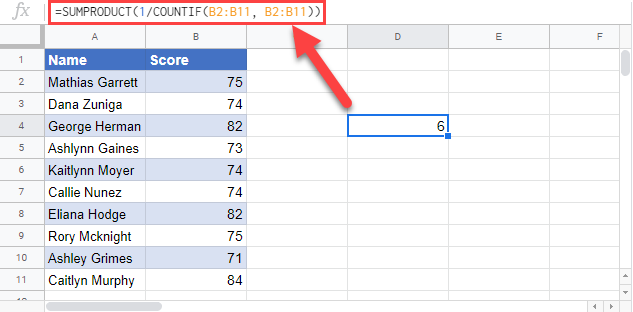Using the same formula, we can get the same result in Google Sheets as well.

### Excel Practice Worksheet

Practice Excel functions and formulas with our 100% free practice worksheets!